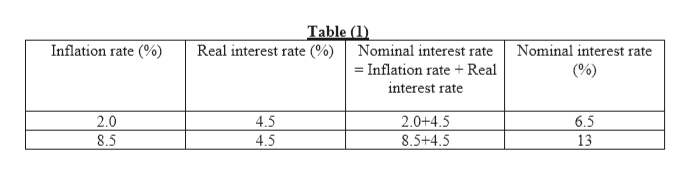# Sean receives a portion of his income from his holdings of interest-bearing U.S. government bonds. The bonds offer a real interest rate of 4.5% per year. The nominal interest rate on the bonds adjusts automatically to account for the inflation rate.The government taxes nominal interest income at a rate of 10%. The following table shows two scenarios: a low-inflation scenario and a high-inflation scenario.Given the real interest rate of 4.5% per year, find the nominal interest rate on Sean's bonds, the after-tax nominal interest rate, and the after-tax real interest rate under each inflation scenario.Inflation RateReal Interest RateNominal Interest RateAfter-Tax Nominal Interest RateAfter-Tax Real Interest Rate(Percent)(Percent)(Percent)(Percent)(Percent)2.04.5   8.54.5   Compared with lower inflation rates, a higher inflation rate will   the after-tax real interest rate when the government taxes nominal interest income. This tends to   saving, thereby   the quantity of investment in the economy and   the economy's long-run growth rate.

Question
102 views

Sean receives a portion of his income from his holdings of interest-bearing U.S. government bonds. The bonds offer a real interest rate of 4.5% per year. The nominal interest rate on the bonds adjusts automatically to account for the inflation rate.

The government taxes nominal interest income at a rate of 10%. The following table shows two scenarios: a low-inflation scenario and a high-inflation scenario.

Given the real interest rate of 4.5% per year, find the nominal interest rate on Sean's bonds, the after-tax nominal interest rate, and the after-tax real interest rate under each inflation scenario.

Inflation Rate

Real Interest Rate

Nominal Interest Rate

After-Tax Nominal Interest Rate

After-Tax Real Interest Rate

(Percent)

(Percent)

(Percent)

(Percent)

(Percent)

2.0 4.5
8.5 4.5

Compared with lower inflation rates, a higher inflation rate will   the after-tax real interest rate when the government taxes nominal interest income. This tends to   saving, thereby   the quantity of investment in the economy and   the economy's long-run growth rate.

check_circle

Step 1

The nominal interest rate can be calculated using following formula-

Step 2

The table (1) below shows the calculation of required nominal interest rate.help_outlineImage TranscriptioncloseTable (1) Real interest rate (% Inflation rate (%) Nominal interest rate Nominal interest rate = Inflation rate+ Real (%) interest rate 2.0+4.5 8.5+4.5 2.0 4.5 6.5 13 4.5 8.5 co fullscreen
Step 3

The after-tax nominal interest rate can be ...

### Want to see the full answer?

See Solution

#### Want to see this answer and more?

Solutions are written by subject experts who are available 24/7. Questions are typically answered within 1 hour.*

See Solution
*Response times may vary by subject and question.
Tagged in